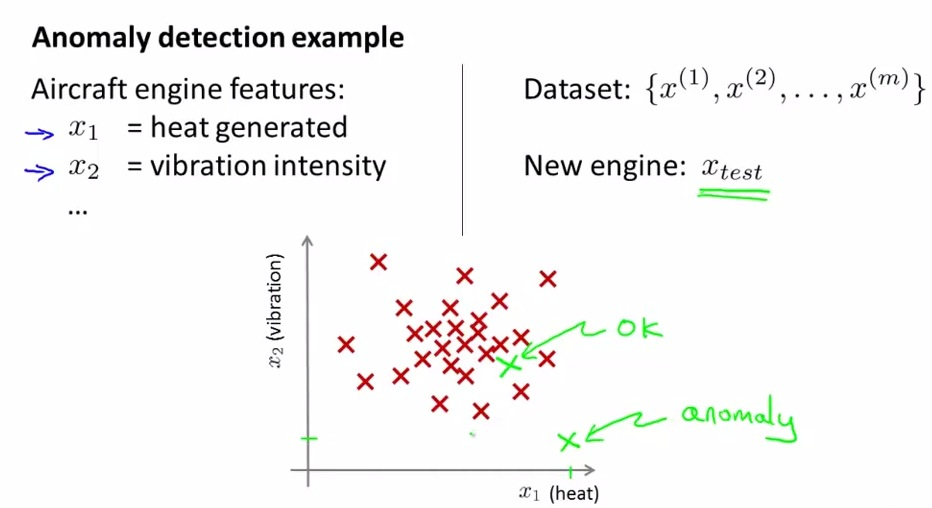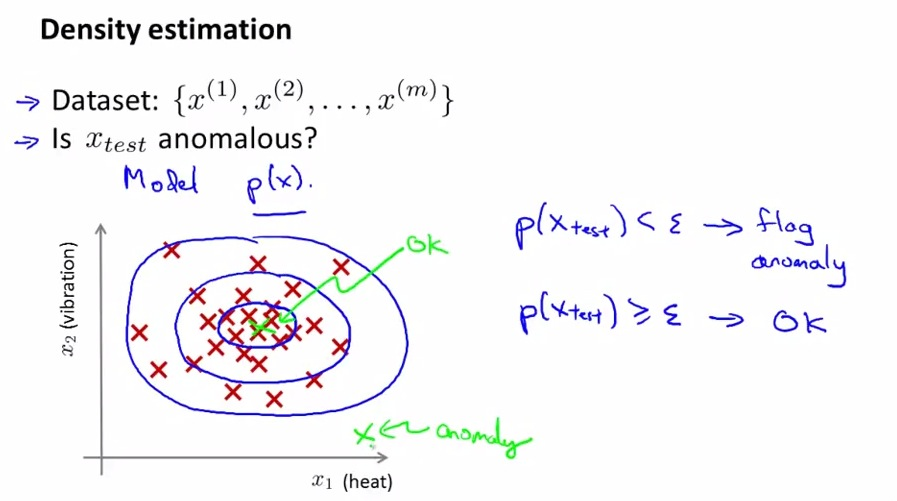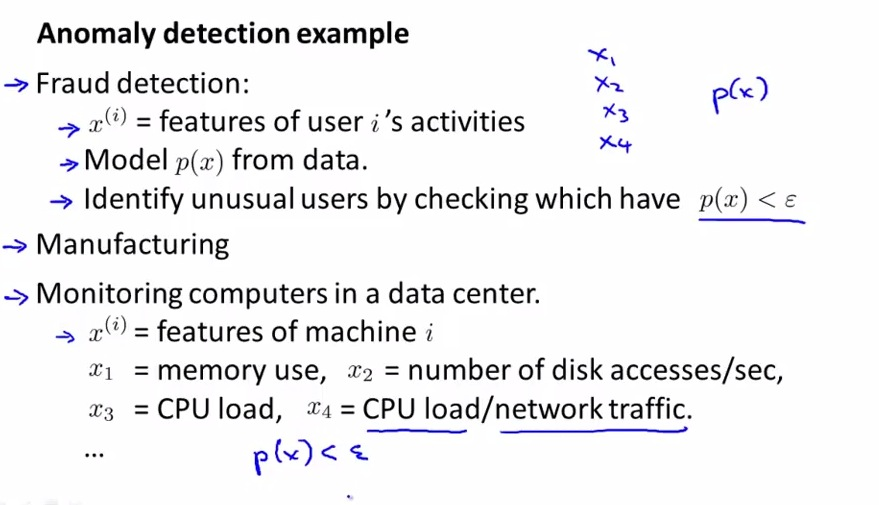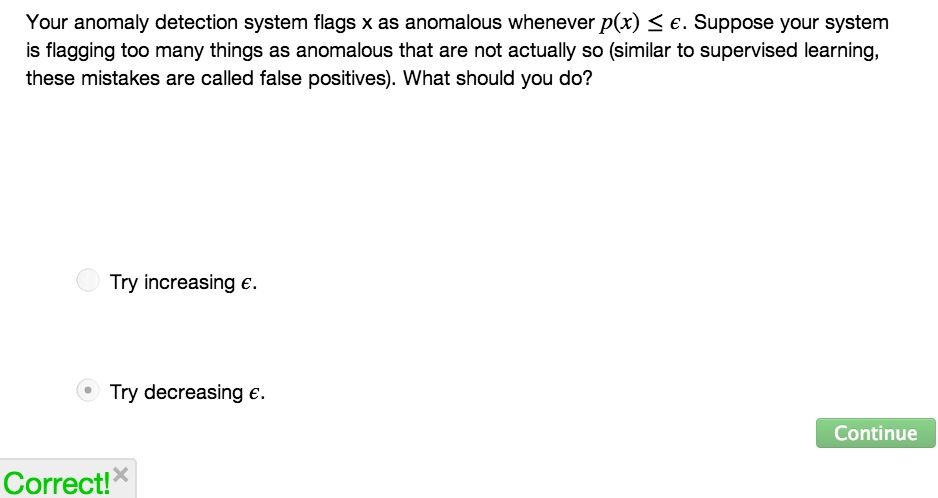• Problem common sighted in unsupervised learning as well as supervised learning• Suppose we have set of features that each of them is going to test the aircraft engine.
• In the example above we just have two set of features that test the engine, so far engines worked well based on the given red mark
• Then we add new engine and make two feature to that engine. It turns out that the test reveal the engine being far away from the rest of the engine.
• This is what known in Machine Learning as Anomaly Detection.• p(x) is the probability of features
• sigma in here denotes some threshold value
• Here we know checking how engine that we tested is less or more than particular threshold value(discussed later, computed by the Gaussian Distribution)• fraud detection user example
• x's = denotes how much user login, typing speed, activity, dll
• technique used by web to analyzed human behaviour
• Manufacturing like jet plane example earlier
• AD on monitoring computers. if one of features is behaving strangely, then there's must be something wrong for the computerSUMMARY
• Next Gaussian distribution and gaussian algorithm for the tools that used for checking anomaly detection